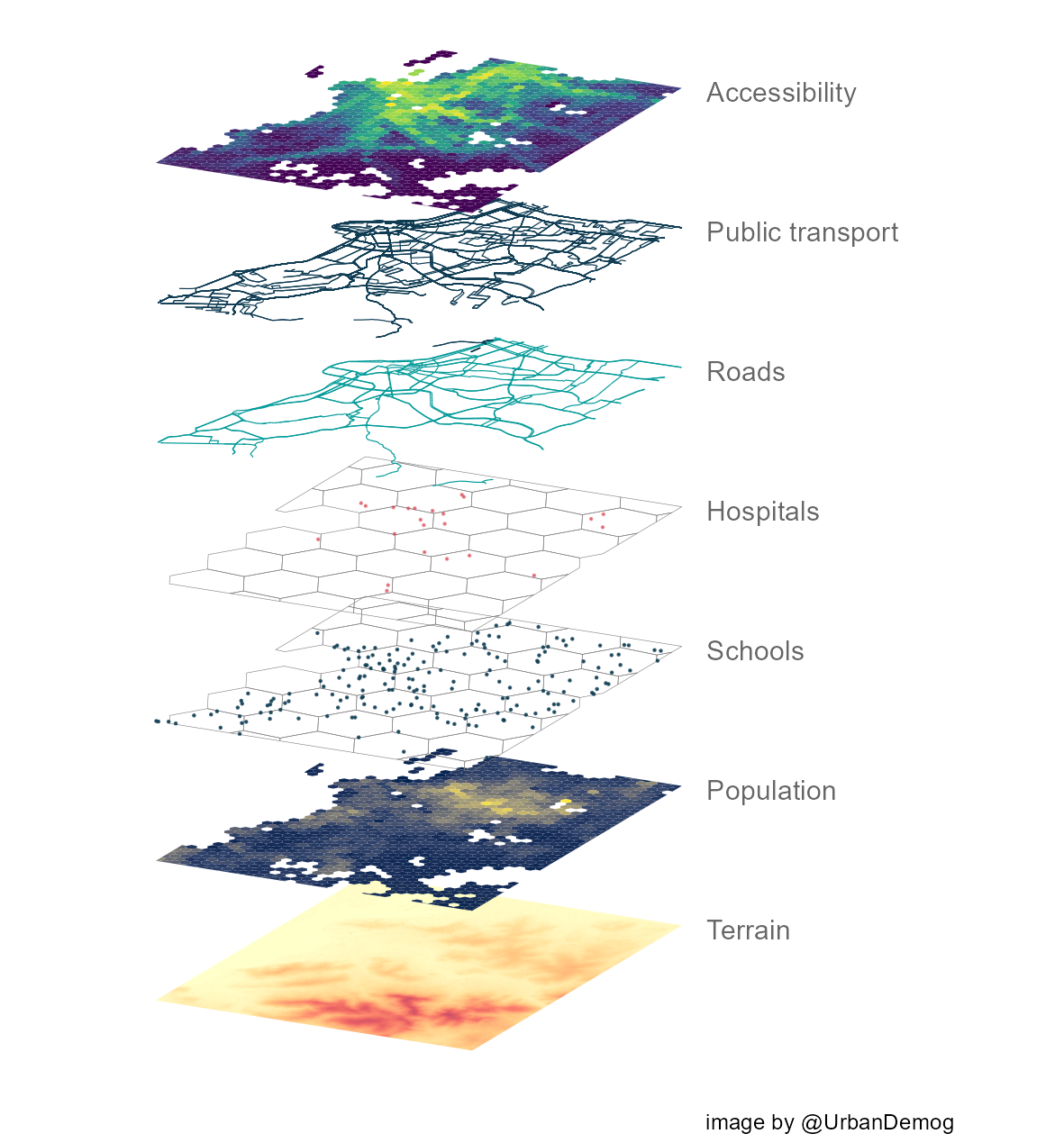## Creating a figure of map layers in R

I like figures of map layers to illustrate the many different types of data sets we combine to do urban and transport modeling. And oftentimes I get obsessed with like making maps that are reproducible with code in `R`. In this post I’ll be sharing a reproducible example showing how to create a figure of stacked maps like this one below.Quick background: In 2014, I was trying to find a way to create map layers in `R`. This was before the `sf` library was created. Most of us were using the `sp` library for handling spatial data and Barry Rowlingson was super helpful, as usual. I used Barry’s suggestion to create a reproducible example so I could use it latter, but then `sf` was created and it completely changed how we do spatial analysis in `R`. Since then, Lauren O’brien proposed a simple way to tilt and stack sf objects and Stefan Jünger created an elegant function to do this. I’ll be using Stefan’s function in my example below.

``````library(easypackages)
easypackages::packages("sf",
"raster",
"stars",
"r5r",
"geobr",
"aopdata",
"gtfs2gps",
"ggplot2",
"osmdata",
"h3jsr",
"viridisLite",
"ggnewscale",
"dplyr",
"magrittr",
prompt = FALSE
)

``````

### Functions to tilt sf

``````rotate_data <- function(data, x_add = 0, y_add = 0) {

shear_matrix <- function(){ matrix(c(2, 1.2, 0, 1), 2, 2) }

rotate_matrix <- function(x){
matrix(c(cos(x), sin(x), -sin(x), cos(x)), 2, 2)
}
data %>%
dplyr::mutate(
)
}

shear_matrix <- function(){ matrix(c(2, 1.2, 0, 1), 2, 2) }

rotate_matrix <- function(x) {
matrix(c(cos(x), sin(x), -sin(x), cos(x)), 2, 2)
}
data %>%
dplyr::mutate(
)
}

``````

We’ll be using a few data sets available from the packages used here. The first thing we need to do is to load the data and crop them to make sure they have the same extent.

``````### get terrain data ----------------

dem <- stars::read_stars(system.file("extdata/poa/poa_elevation.tif", package = "r5r"))
dem <- st_as_sf(dem)

# crop
bbox <- st_bbox(dem)

### get public transport network data ----------------

gtfs <- gtfs2gps::read_gtfs( system.file("extdata/poa/poa.zip", package = "r5r") )
gtfs <- gtfs2gps::gtfs_shapes_as_sf(gtfs)

# crop
gtfs <- gtfs[bbox,]
gtfs <- st_crop(gtfs, bbox)
plot(gtfs['shape_id'])

### get OSM data ----------------

value = c("motorway", "primary","secondary")) %>% osmdata_sf()

# crop

### get H3 hexagonal grid ----------------

# get poa muni and hex ids
poa <- read_municipality(code_muni = 4314902 )
hex_ids <- h3jsr::polyfill(poa, res = 7, simple = TRUE)

# pass h3 ids to return the hexagonal grid
hex_grid <- h3jsr::h3_to_polygon(hex_ids, simple = FALSE)
plot(hex_grid)

# crop
hex_grid <- hex_grid[bbox,]
hex <- st_crop(hex_grid, bbox)
plot(hex)

### get land use data from AOP project ----------------

landuse <- aopdata::read_access(city = 'poa', geometry = T, mode='public_transport')

# crop
landuse <- landuse[bbox,]
landuse <- st_crop(landuse, bbox)
plot(landuse['CMATT30'])

# hospitals
# generate one point per hospital in corresponding hex cells
df_temp <- subset(landuse, S004>0)
hospitals <- st_sample(x = df_temp, df_temp\$S004, by_polygon = T)
hospitals <- st_sf(hospitals)
hospitals\$geometry <- st_geometry(hospitals)
hospitals\$hospitals <- NULL
hospitals <- st_sf(hospitals)
plot(hospitals)

# schools
# generate one point per schools in corresponding hex cells
df_temp <- subset(landuse, E001>0)
schools <- st_sample(x = df_temp, df_temp\$E001, by_polygon = T)
schools <- st_sf(schools)
schools\$geometry <- st_geometry(schools)
schools\$schools <- NULL
schools <- st_sf(schools)
plot(schools)

``````

### Plot

``````### plot  ----------------

# annotate parameters
x = -141.25
color = 'gray40'

temp1 <- ggplot() +

# terrain
geom_sf(data = dem %>% rotate_data(), aes(fill=poa_elevation.tif), color=NA, show.legend = FALSE) +
scale_fill_distiller(palette = "YlOrRd", direction = 1) +
annotate("text", label='Terrain', x=x, y= -8.0, hjust = 0, color=color) +
labs(caption = "image by @UrbanDemog")

temp2 <- temp1 +

# pop income
new_scale_fill() +
new_scale_color() +
geom_sf(data = subset(landuse,P001>0) %>% rotate_data(y_add = .1), aes(fill=R001), color=NA, show.legend = FALSE) +
scale_fill_viridis_c(option = 'E') +
annotate("text", label='Population', x=x, y= -7.9, hjust = 0, color=color) +

# schools
geom_sf(data = hex %>% rotate_data(y_add = .2), color='gray50', fill=NA, size=.1) +
geom_sf(data = schools %>% rotate_data(y_add = .2), color='#0f3c53', size=.1, alpha=.8) +
annotate("text", label='Schools', x=x, y= -7.8, hjust = 0, color=color) +

# hospitals
geom_sf(data = hex %>% rotate_data(y_add = .3), color='gray50', fill=NA, size=.1) +
geom_sf(data = hospitals %>% rotate_data(y_add = .3), color='#d5303e', size=.1, alpha=.5) +
annotate("text", label='Hospitals', x=x, y= -7.7, hjust = 0, color=color) +

# OSM
annotate("text", label='Roads', x=x, y= -7.6, hjust = 0, color=color) +

# public transport
geom_sf(data = gtfs %>% rotate_data(y_add = .5), color='#0f3c53', size=.2) +
annotate("text", label='Public transport', x=x, y= -7.5, hjust = 0, color=color) +

# accessibility
new_scale_fill() +
new_scale_color() +
geom_sf(data = subset(landuse, P001>0) %>% rotate_data(y_add = .6), aes(fill=CMATT30), color=NA, show.legend = FALSE) +
scale_fill_viridis_c(direction = 1, option = 'viridis' ) +
theme(legend.position = "none") +
annotate("text", label='Accessibility', x=x, y= -7.4, hjust = 0, color=color) +
theme_void() +
scale_x_continuous(limits = c(-141.65, -141.1))

# save plot
ggsave(plot = temp2, filename = 'map_layers.png',
dpi=200, width = 15, height = 16, units='cm')

``````